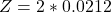Question

You illuminate a slit with a width of 77.7 μm with a light of wavelength 721 nm and observe the resulting diffraction pattern on a screen that is situated 2.83 m from the slit. What is the width, in centimeters, of the pattern’s central maximum

1.haidang

The width is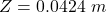Explanation:

From the question we are told that

The width of the slit is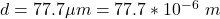The wavelength of the light is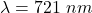The position of the screen is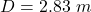Generally angle at which the first minimum  of the interference pattern the  light occurs  is mathematically  represented as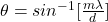Where m which is the order of the interference is 1

substituting values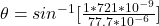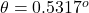Now the width of first minimum  of the interference pattern is mathematically evaluated as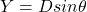substituting values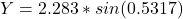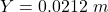Now the width of  the  pattern’s central maximum is mathematically evaluated as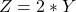substituting values